# What is Graph in Data Structures?

We have started studying graphs when we were in 5th or 6th standard, and now studying the concept of the graph in the data structure is really confusing. You might be wondering What is a Graph in Data Structure, what are the various applications of a graph, why are we studying it in Data Structure, and many more.

Don't worry here in this blog you will get all the answers for your queries related to graphs like what is a graph, types of graphs, how graphs are used in the data structure, how they are represented in computer memory and some examples of real-life applications of the graph.

## What is Data Structure?

Let me first introduce you to the definition of Data Structure before moving on to Graphs in Data Structure. Data Structure is the organization of data in a particular manner so that the data can be easily understandable. We can see few examples of Data Structures like Arrays, Strings, Linked Lists, etc. And the graph is also a very common example of data structure.

You can use Data Structure on any kind of Operating System, after Windows, Linux is the Operating System that is gaining huge demand. To install Linux on your system you can refer to the blog How to Install Arch Linux.

## What is Graph in Data Structure?

A graph is a non-linear type of data structure that consists of a finite set of nodes and edges. The interconnected objects are represented as nodes and the connection between them is represented as an edge. Mathematically, a graph is a pair of vertices V and edges E, identified with a unique pair [u,v] of nodes in V, denoted by e = [u,v].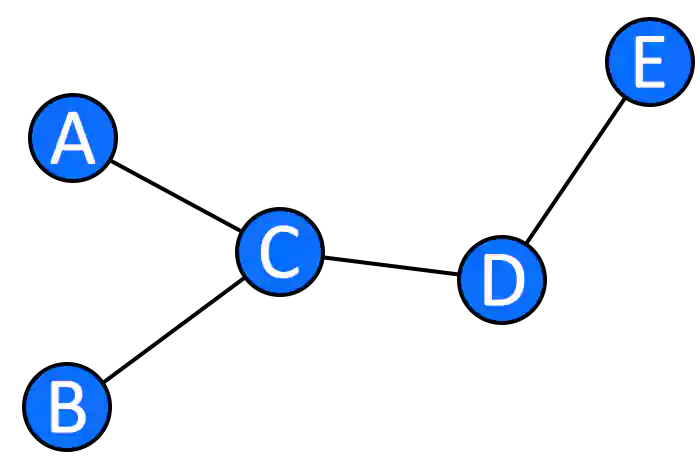## Representation of a Graph in Data Structure

Representation of the graph means how the data is stored in the computer memory. To represent a graph in computer memory, we need vertices and edges of a graph. There are three ways in which graphs can be represented:

Adjacency Matrix is a square matrix used to represent graphs having a finite number of nodes. It represents the adjacent nodes to each other. We have to construct a n x n matrix, if a node is connected to another node then a[i], a[j] = 1 else a[i], a[j] are equal to zero. If we have a graph with 10 nodes, we have to construct a matrix of 10 x 10.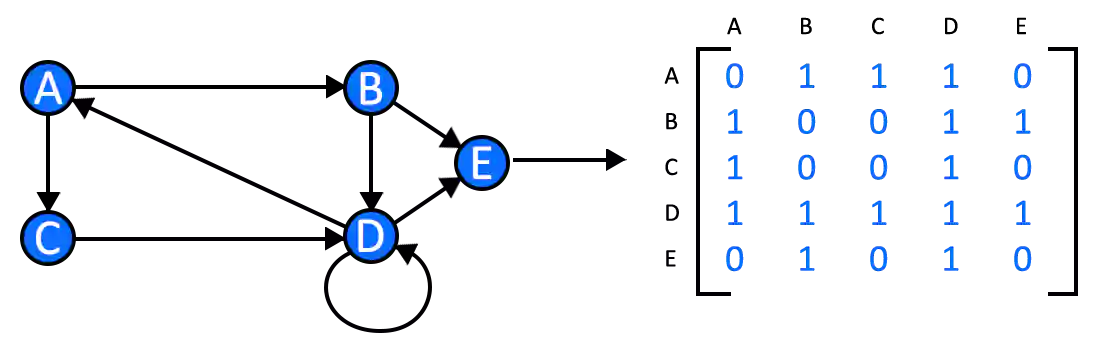• It is easy to check which nodes are connected other one and which node is not connected.
• Adjacency Matrices are easy to implement and follow.

• It requires a large memory complexity.
• It is helpful only when the number of nodes are less.
• ### Incidence Matrix

Incidence Matrix is represented by constructing a matrix of size (number of vertices x number of edges), the number of vertices is represented by rows and the number of edges is represented by columns. This matrix can be square or rectangle.

We can have 0, 1, or -1 as the values of the Incidence Matrix. 0 is used to represent an edge which is not connected to any vertex. 1 is used to represent the edge which is connected to the outgoing vertex. -1 is used to represent the edge that is connected to the incoming vertex.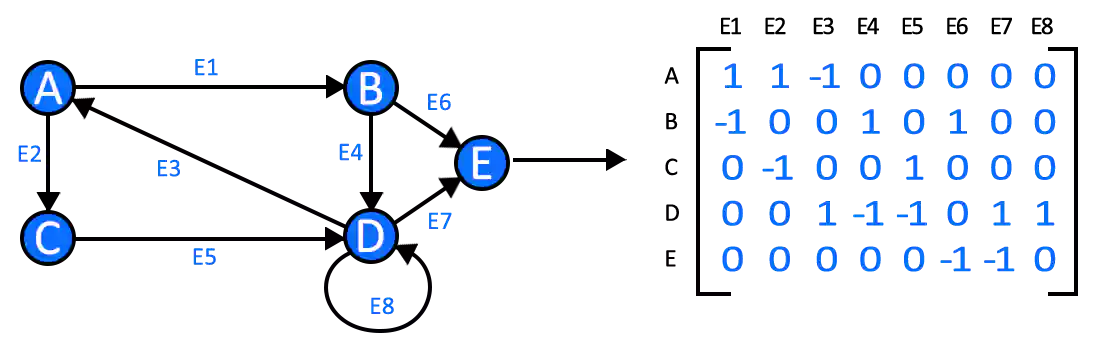• No advance structure is needed to represent the graph.
• This representation is easy to understand and implement.

• Consumes a large amount of space.

Adjacency List is the list representation of the graph. Every vertex of the graph contains a list of its neighbouring vertices.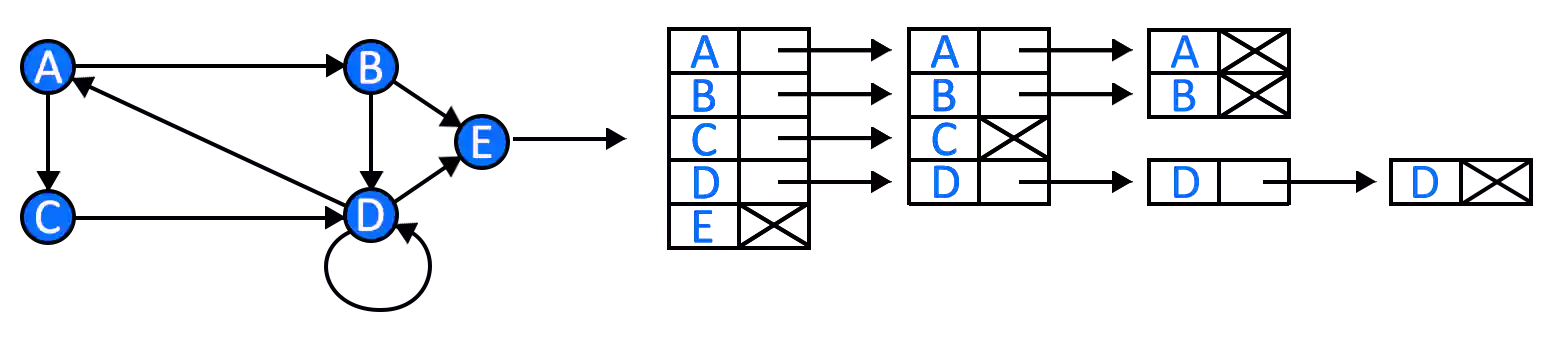• It saves a lot of memory in the system.
• Insertion and deletion of nodes are easy in the Adjacency List.
• Allow us to compactly represent the graph.

• Adjacency lists are useful when there is a large graph, but when the graph is small, a lot of memory space is required to represent the graph.

## Types of Graphs

There are many types of Graphs in Data Structure. Some of the commonly used graphs are:

1. Finite Graph

A graph having a finite number of vertices and edges is called a Finite Graph. Most of the graphs that we will discuss are finite.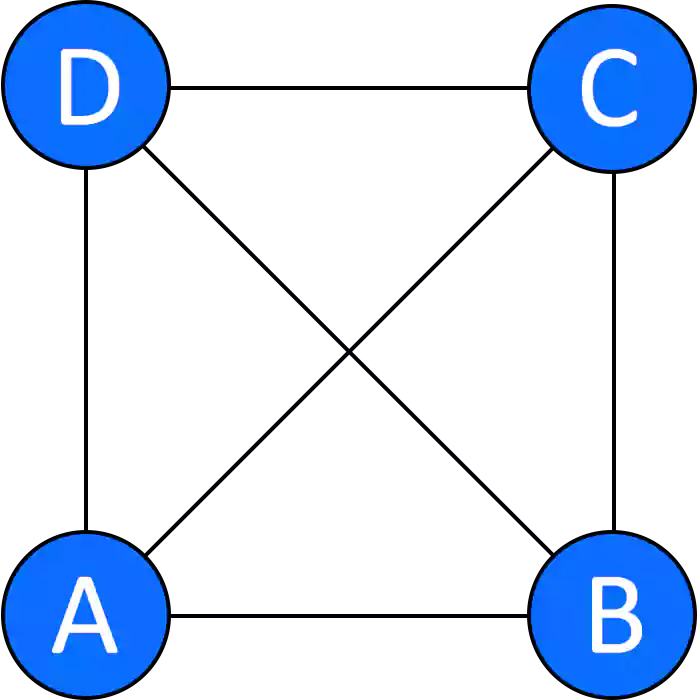1. Infinite Graph

A graph in which there is no end of the number of vertices and edges is called an Infinite Graph.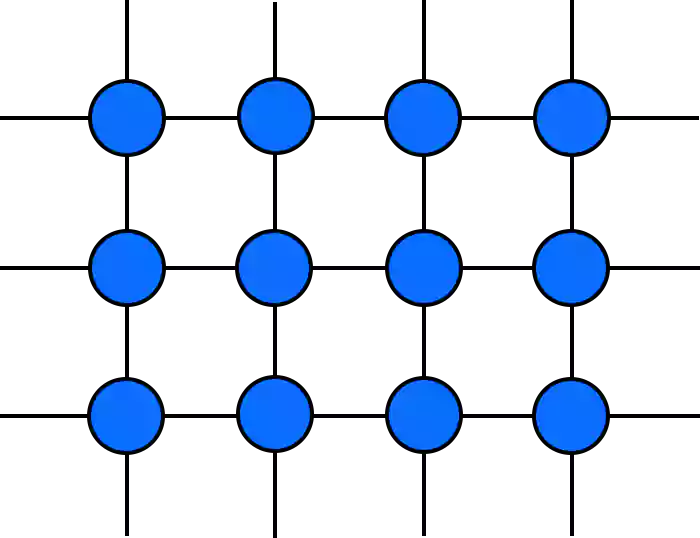1. Directed Graph

A graph where a set of vertices are directed to each other, giving the path to follow. It is also known as Digraph.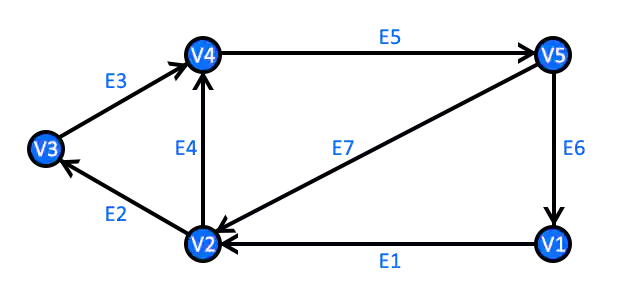1. Undirected Graph

Graphs in which directions are not shown can follow the path from one node to another as we want. This type of graph is known as an Undirected Graph.

1. Weighted Graph

The graph whose edges or paths are numerically weighted are known as Weighted Graphs. The weighted values are generally positive.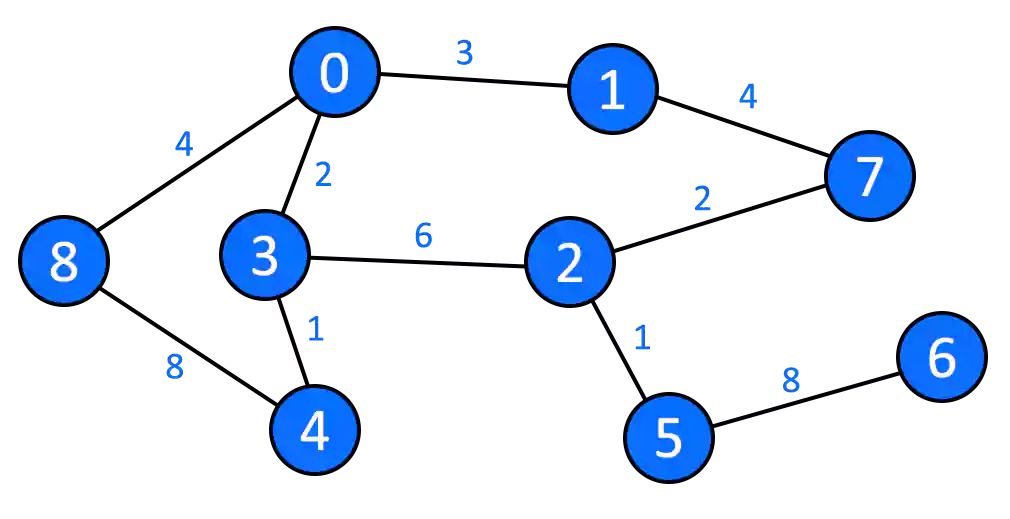1. Unweighted Graph

The graph which is not weighted is an Unweighted Graph. In other words, the graph whose edges or paths do not have any numerical weighted value are known as unweighted graphs. By default, all the graphs are unweighted, we give them numerically positive values if we need them.

1. Null Graph

A graph with a number of vertices but does not have any edge connecting those vertices is a Null Graph.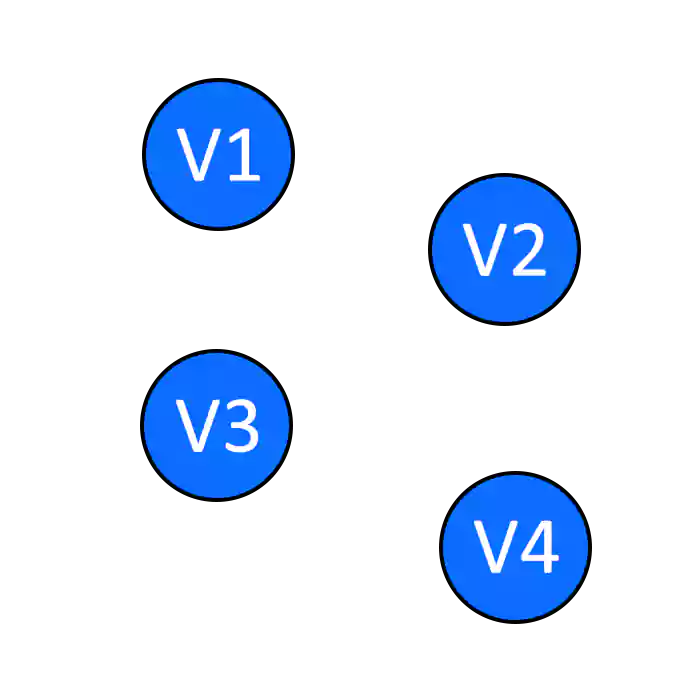1. Trivial Graph

A graph with only one vertex and zero edges is called a Trivial Graph.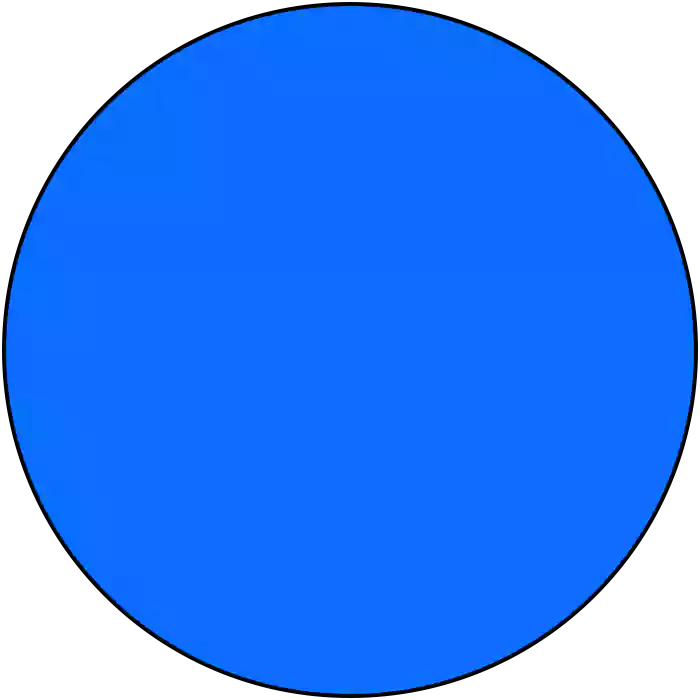1. Complete Graph

A graph whose all the vertices are connected to each other is a Complete Graph. A complete graph is also known as a Full Graph.1. Cyclic Graph

A graph consisting of n number of vertices where n>3, which are connected to each other making a cycle is called a Cyclic Graph.## Examples of Graphs

You are wondering that there are different types of graphs but what are the practical applications of the graph in the data structure. You will be surprised to know that Graph in Data Structure has amazing uses in our daily life.

### Computer Science

Graphs are used in a wide range in the field of computer science. They are used in the study of various algorithms, used to represent the flow of computation, a network of communication, used in social graphs, and many more areas.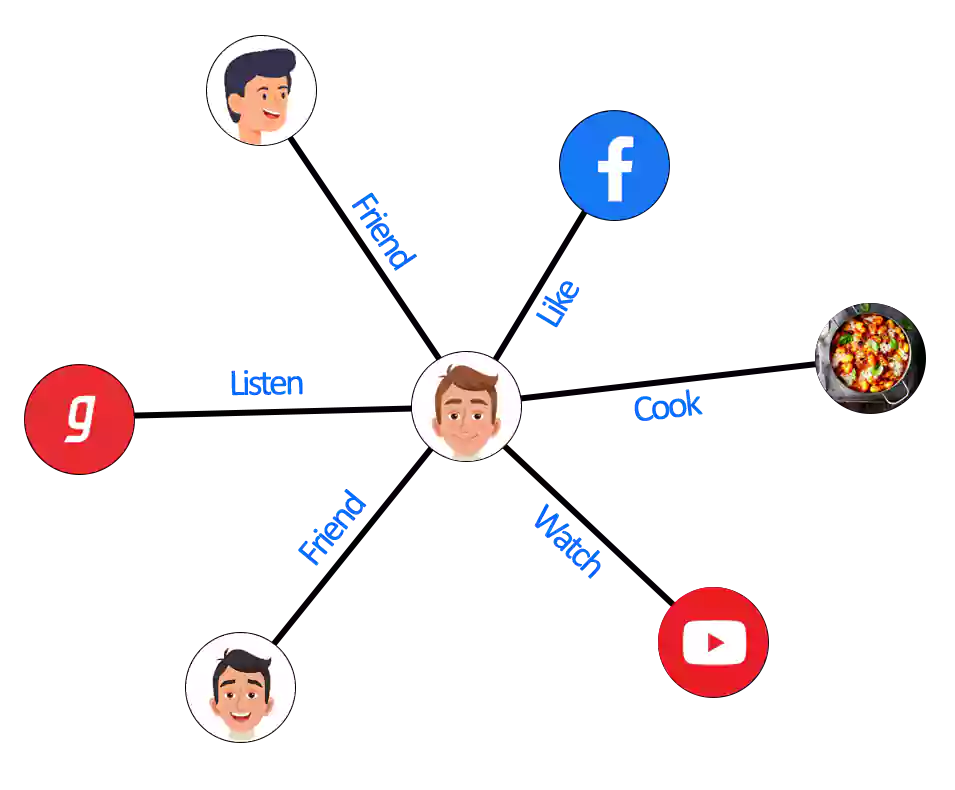Maps are an interconnection of roads, the point where the two roads meet is a vertex and the road is an edge. Google uses graphs to represent the complicated structure of the globe effectively. In Google Maps graphs are used to find the shortest path from the place of origin to the place of destination.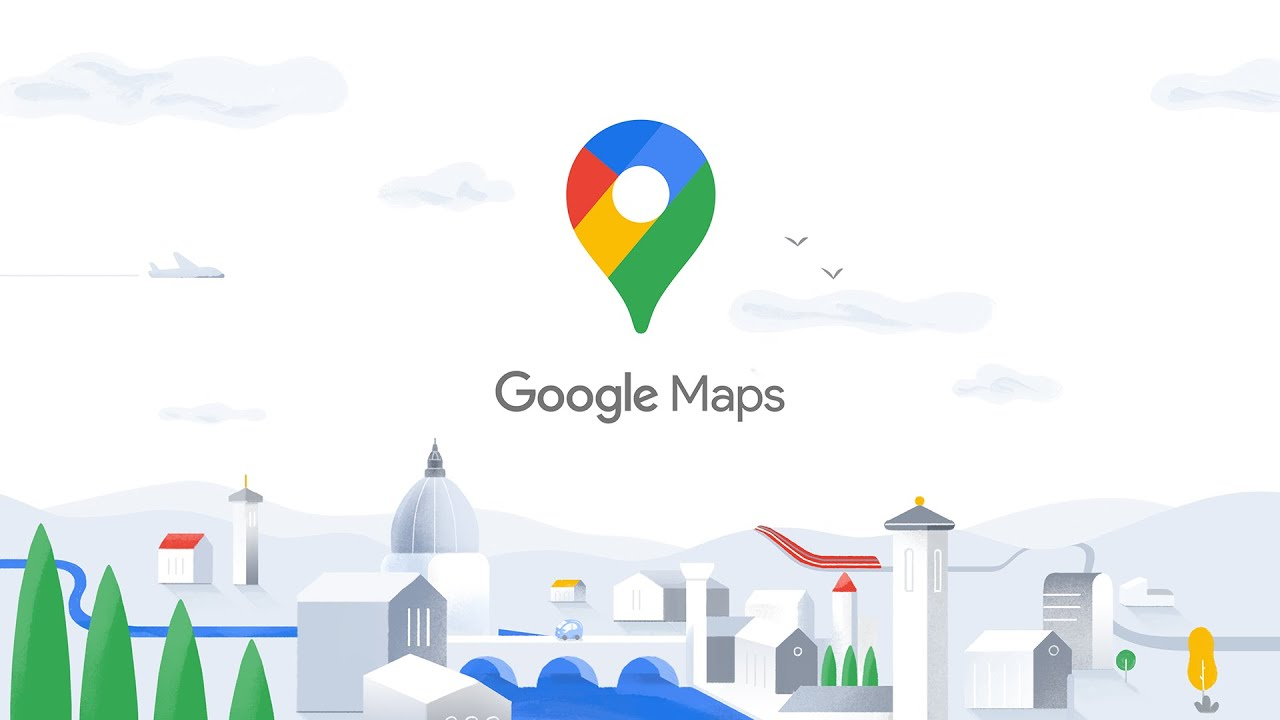Facebook is a collection of nodes and edges. When you send a friend request to a person, you and the person are the nodes and the connection between you two is an edge. When you post a picture, join a group, every action you do on the application is creating a relationship between you and the action you performed. Facebook is made of a big collection of nodes and edges, using graphs on a larger level.### Recommendation Engine

If the concept of the graph in data structure never came, then you will never see any recommendations. Here I am giving the example of your location, you have got recommendations of a restaurant near you or any place you are searching for no matter in which part of the world you are. You might have received notifications from Google for receiving feedback on the location you have just visited. Have you ever thought about how Google knows everything? This is because your location is traced with the graphs. The MAC (Media Access Control) Address of your system helps in tracking the location of your system.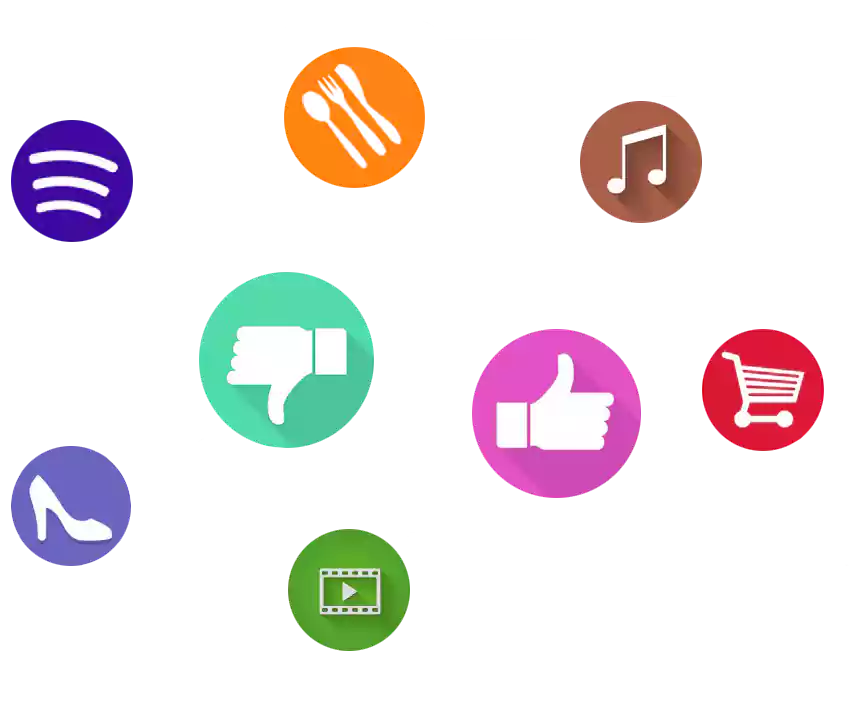## Conclusion

I have tried to cover as much information as I can. If you feel something is left unmentioned, you have any queries or suggestions then you can freely contact us in the comment section below. We will love to have feedback from you.

How To Install Arch Linux 2023 [Installation Guide] | Techofide

Boot Menu Option Keys For All Computers and Laptops | [Updated List 2021] | Techofide

## Subscribe Us

* indicates required

#### Dhairya

I am a student of Bachelor of Computer Applications. I love to research and write … readmore